# HSSlive: Plus One & Plus Two Notes & Solutions for Kerala State Board

## BSEB Class 12 Physics Moving Charges and Magnetism Textbook Solutions PDF: Download Bihar Board STD 12th Physics Moving Charges and Magnetism Book AnswersBSEB Class 12 Physics Moving Charges and Magnetism Textbook Solutions PDF: Download Bihar Board STD 12th Physics Moving Charges and Magnetism Book Answers

BSEB Class 12th Physics Moving Charges and Magnetism Textbooks Solutions and answers for students are now available in pdf format. Bihar Board Class 12th Physics Moving Charges and Magnetism Book answers and solutions are one of the most important study materials for any student. The Bihar Board Class 12th Physics Moving Charges and Magnetism books are published by the Bihar Board Publishers. These Bihar Board Class 12th Physics Moving Charges and Magnetism textbooks are prepared by a group of expert faculty members. Students can download these BSEB STD 12th Physics Moving Charges and Magnetism book solutions pdf online from this page.

## Bihar Board Class 12th Physics Moving Charges and Magnetism Books Solutions

 Board BSEB Materials Textbook Solutions/Guide Format DOC/PDF Class 12th Subject Physics Moving Charges and Magnetism Chapters All Provider Hsslive

## How to download Bihar Board Class 12th Physics Moving Charges and Magnetism Textbook Solutions Answers PDF Online?

2. Click on the Bihar Board Class 12th Physics Moving Charges and Magnetism Answers.
3. Look for your Bihar Board STD 12th Physics Moving Charges and Magnetism Textbooks PDF.
4. Now download or read the Bihar Board Class 12th Physics Moving Charges and Magnetism Textbook Solutions for PDF Free.

Find below the list of all BSEB Class 12th Physics Moving Charges and Magnetism Textbook Solutions for PDF’s for you to download and prepare for the upcoming exams:

Question 1. A circular coil of wire consisting of 100 turns, each of radius 8.0 cm carries a current of 0.40 A. What is the magnitude of the magnetic field B at the centre of the coil? Answer: Here, n = number of turns in the coil = 100 r = radius of each turn of the coil = 8.0 cm = 8 x 10-2 m. I = current in the circular coil = 0.40 A. Let B = magnitude of magnetic field at the centre of coil = ? Using the relation, B = 𝜇04𝜋⋅2𝜋nlr we get B = 10-7 x 2×3.14×100×0.408×10−2 = 3.14 x 10-4T

Question 2. A long straight wire carries a current of 35 A. What is the magnitude of the field Bata point 20 cm from the wire? Answer: Here, I = current flowing in the straight wire = 35 A. a = distance of the point from the straight wire = 20 cm B = magnitude of the magnetic field = ? Using the relation, B = 𝜇04𝜋⋅2𝜋nlr weget B = 10-7 x 2×3520×10−2 = 3.5 x 10-5 T

Question 3. A long straight wire in the horizontal plane carries a current of 50 A in north to south direction. Give the magnitude and direction of B at a point 2.5 m east of the wire. Answer: Here, I = current in the long wire from north to south direction = 50 A. a = perpendicular distance of the point (P say) from the wire = 2.5 m. Let B be the magnitude of the field at P. .’. Using the relation, B = 𝜇04𝜋⋅2𝜋nlr we get B = 10-7 x 2×502.5 = 0.4 x 10-5 T = 4 x 10-6 TDirection of B→ : Applying right hand thumb rule, we find that the direction of B→ at the observation point is towards the east i.e., If we assume that NS direction lies in the plane of paper, then B→ will be perpendicular to the plane of paper and in the vertically upward direction,

Question 4. A horizontal overhead power line carries a current of 90 A in east to west direction. What is the magnitude and direction of the magnetic field due to the current 1.5 m below the line? Answer: Here, I = current in the power line = 90 A in east to west direction.a = 1.5 m. Let B = magnetic field at point P (say) at a distance 1.5 m below the line. ∴ Using the relation, B = 𝜇04𝜋⋅2𝜋nlr, we get B = 10-7 x 2×901.5 = 1.2 x 10-5 T

Direction of B→ : According to right hand thumb rule, the direction of the magnetic field is towards the south at the point of observation.

Question 5. What is the magnitude of magnetic force per unit length on a wire carrying a current of 8 A and making an angle of 30° with the directiorf of a uniform magnetic field at 0.15 T? Answer: Here, I = Current in the wire = 8A. θ = angle made bv the wire with the uniform magnetic field B→ = 30° B = 0.15 T Let F be the magnetic force per unit length on the wire = ?

∴ Using equation F = BI sin θ , we get F = 0.15 x 8 x sin 30° (Nm-1) = 0.15 x 8 x 12 = 0.15 x 4 = 0.60 Nm-1.

Question 6. A 3.0 cm wire carrying a current of 10 A is placed inside a solenoid perpendicular to its axis. The magnetic field inside the solenoid is given to be 0.27 T. What is the magnetic force on the wire? Answer: Here, l = length of the wire = 3.0 cm = 3 x 10-2 m. I = current in the wire = 10 A. B = magnetic field inside the solenoid = 0.27 T 0 = angle made by the wire with the axis of the solenoid = 90° Let F = magnitude of the magnetic force on the wire. ∴ Using eqn, F = BIZ sin θ, we get F = BI l sin 90° = BIl = 0.27 x 10 x 3 x 10-2 = 8.1 x 10-2 N. Direction of F: Direction of F→ is found using Fleming’s left hand rule.

Question 7. Two long and parallel straight wires A and B carrying currents of 8.0 A and 5.0 A in the same direction are separated by a distance of 4.0 cm. Estimate the force on a 10 cm section of wire A. Answer: Here, Let I1, and I2 be the currents flowing in the straight long and parallel wires A and B respectively. ∴ I1 = 8.0 A, I2 = 5.0 A flowing in the same direction. r = distance between A and B = 4.0 cm = 4 x 10-2 m. If F’ be the force per unit length on wire A, then using F’ = 𝜇04𝜋⋅2𝜋nlr we get F’ = 10-7 x 2×8×54×10−2 Nm-1 = 20 x 10-5 Nm-1. If F be the force on a section of length 10 cm of wire A, then F = F’ x l (Here, l = 10 x 10-2 m) = 20 x 10-5 x 10 x 10-2 N = 2 x 10-5N. Direction of F→ : As the current in the two parallel wires is flowing in the same direction, so the force will be attractive normal to A towards B.

Question 8. A closely wound solenoid 80 cm long has 5 layers of windings of 400 turns each. The diameter of the solenoid is 1.8 cm. If the current carried is 8.0 A, estimate the magnitude of B inside the solenoid near its centre. Answer: Here, l = length of the long solenoid = 80 cm = 80 x 10-2 m. n’ = no. of turns in each layer = 400 n = no. of layers in the solenoid =5. N = total number of turns in the solenoid = n’n = 400 x 5 = 2000. I = current flowing in the solenoid = 8.0 A. D = Diameter of the solenoid = 1.8 cm = 1.8 x 10-2 m. Let B be the magnitude of the magnetic field inside the solenoid near its centre = ? 𝜇0NI𝑙 = 4𝜋×10−7×2000×880×10−2 T = 8π x 10-3 T = 2.5 x 10-2 T.

Question 9. A square coil of side 10 cm consists of 20 turns and carries a current of 12 A. The coil is suspended vertically and the normal to the plane of the coil makes an angle of 30° with the direction of a uniform horizontal magnetic field of magnitude 0.80 T. What is the magnitude of torque experienced by the coil? Answer: Here, a = side of square coil = 10 cm = 10 x 10-2 m. N = total number of turns in the coil = 20 I = current flowing in the coil = 12 A. B = magnitude of uniform magnetic field = 0.80 T. θ = angle made by the normal to the plane of coil with B = 30° ∴ A = area of coil = a2 = (10 x 10-2) m2. τ = magnitude of the torque experienced by the coil = ?

Using the formula, τ = NIA B sin θ, we get τ = 20 x 12 x (10 x 10-2)2 x 0.80 x sin 30° = 20 x 12 x 10-2 x 0.80 x 12 = 0.96 Nm.

Question 10. Two moving coil meters, M1 and M2 have the following particulars: R1 = 10 Ω, N1 = 30, A1 = 3.6 x 10-3 m2, B1 = 0.25 T R2 = 14 Ω, N2 = 42, A2 = 1.8 x 10-3 m2, B2 = 0.50 T (The spring constants are identical for the two meters). Determine the ratio of (a) current sensitivity and (b) voltage sensitivity of M2 and M1. Answer: Here, R1 = 10 Ω, N1 = 30, A1 = 3.6 x 10-3 m2, B1 = 0.25 T for coil M1 R2 = 14 Ω, N2 = 42, A2 = 1.8 x 103 m2, B2 = 0.50 T for coil M2. We know that current sensitivity and voltage sensitivity are given by the formulae. Current sensitivity = 𝑁𝐵𝐴𝐾 and Voltage sensitivity = 𝑁𝐵𝐴𝐾𝑅 Here k1 = k2 for the two coils = k (say) ∴ Current sensitivity for M1 is given by = N1 B1A1/k and for M2 = N2 B2 A2k

(a) Current resistivity ratio for M2 and M1 is given byNow voltage resistivity for M1 is = 𝑁1𝐵1𝐴1𝑘𝑅1 and for M2 = N2 B2 A2kR2

(b) Voltage sensitivity ratio for M2 and M1 is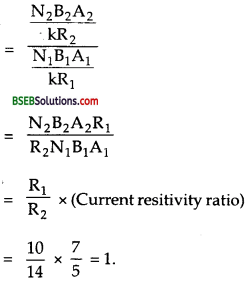Question 11. In a chamber, a uniform magnetic field of 6.5 G (1 G = 10-4 T) is maintained. An electron is shot into the field with a speed of 4.8 x 106 ms-1 normal to the field. Explain why the path of the electron is a circle. Determine the radius of the circular orbit, (e = 1.6 x 10-19 C, me = 9.1 x 10-31 kg) Answer: Here, B = 6.5 G = 6.5 x 10-4 T = uniform magnetic field. υ = speed of the electron shot normal to E→ = 4.8 x 106 ms-1 e = charge on an electron = 1.6 x 10-19 C. me = mass of an electron, =9.1 x 1031kg. θ = 90° C If r be the radius of the circular orbit, then the force F on the moving electron due to the magnetic field is given by F = e υ B sin θ . The direction of the force is perpendicular to v→ as well as to B→ , so this force will only change the direction of motion of the electron without affecting its speed and this force provides the necessarily centrepetal force to the moving electron, so the electron will move on the circular path. If r be the radius of the circular path followed by the electron, then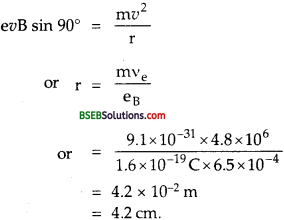Question 12. In Exercise 4.11 obtain the frequency of revolution of the electron in its circular orbit. Does the answer depend on the speed of the electron? Explain. Answer: Let v = Frequency of revolution of electron in the orbit = ? Again using the formula,From equation (1), we see that the frequency of revolution of the electron does not have the speed of the electron, so v does not depend on the speed of the electron.

Question 13. (a) A circular coil of 30 turns and radius 8.0 cm carrying a current of 6.0 A is suspended vertically in a uniform horizontal magnetic field of magnetude 1.0 T. The field lines make an angle of 60° with the normal of the coil. Calculate the magnitude of the counter torque that must be applied to prevent the coil from turning.

(b) Would your answer change, if the circular coil in (a) were replaced by a planar coil of some irregular shape that encloses the same area? (All other particulars are also unaltered.) Answer: Here, N = no. of turns in the circular coil = 30 r = radius of the ciruclar coil = 8.0 cm = 8 x 10-2 m. I = current in the circular coil = 6.0 A. B = uniform horizontal magnetic field = 1.0 T. θ = angle between B→ and the normal to the plane of coil = 60° ∴ area of circular coil, A = πr-2 = 3.14 x (8 x 10-2)2 m2 = 3.14 x 64 x 10-4 m2 = 2.01 x 10-2 m2.

(a) Magnitude of torque acting on the current carrying coil due to the magnetic field is given by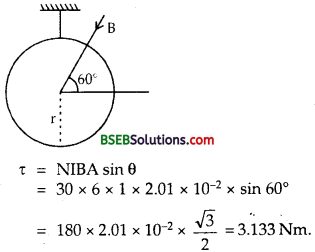To prevent the coil from rotating, a torque equal and opposite to x has to be applied ∴ Torque required = τ = 3.133 Nm.

(b) No. Since the torque on a planar loop is independent of its shape in case the area of the loop is kept same; hence the torque will remain unchanged.

Question 14. Two concentric circular coils X and Y of radii 16 cm and 10 cm, respectively, lie in the same vertical plane containing the north to south direction. Coil X has 20 turns and carries a current of 16 A; coil Y has 25 turns and carries a current of 18 A. The sense of the current in X is anticlockwise, and clockwise in Y, for an observer looking at the coils facing west. Give the magnitude and direction of the net magnetic field due to the coils at their centre. Answer: For coil X: Here, a = radius of coil = 16 cm = 16 x 10-2 m. n = no. of turns in the coil = 20 I = current in the coil = 16 A (anticlockwise) Bx = The magnetic field at the centre at the coil X = ?Using formula, B = 𝜇04𝜋⋅2𝜋𝑛𝐼𝑄 …….(1)

Weget B = 𝜇04𝜋⋅2𝜋𝑛𝐼9 = 10−7×2×𝜋×20×1616×10−2 = 4π x 10-4 T For an observer looking at the coil X facing west, the current is flowing in the anticlockwise direction, so the direction of B is towards east.

For coil Y: Here, a = 10 cm = 10 x 10-2 m n = 25 I = 18 A (clockwise) By = magnetic field at the centre of the coil Y = ? Using eqn (1), we get By = 𝜇04𝜋⋅2𝜋nIa = 10-7 x 2𝜋×25×1810×10−2 T = 9π x 10-4T. For an observer looking at the coil Y facing west, the current is flowing in the clockwise direction, so the direction of field By is towards west. ‘ Now as Bx and By act along the same line in the opposite direction, so the resultant magnetic field will act in the direction of bigger vector. So if E→ be the net magnetic field due to the two coils at their centre, then E→ = ry→ + rx→ = 9π x 10-4 (west) + 471 x 10-4 (east) = (9π x 10-4 – 4π x 10-4) west = 5π x 10-4 T towards west = 5 x 3.14 x 10-4 T towards west = 1.6 x 10-4T towards west.

Question 15. A magnetic field of 100 G (1G = 10-4 T) is required which is uniform in a region of linear dimension about 10 cm and area of cross-section about 10-3 m2. The maximum current-carrying capacity of a given coil of wire is 15 A and the number of turns per unit length that can be wound round a core is at most 1000 turns m-1. Suggest some appropriate design particulars of a solenoid for the required purpose. Assume the core is not ferromagnetic. Answer: Here, B = magnetic field = 100 G = 100 x 10-4 = 10-2 T, Imax maximum current carried by the coil = 15 A. n = number of turns per unit length = 1000 turns m-1 = 10 turns m-1 = 10 turns / cm. l = length of linear region = 10 cm A = area of cross-section = 10-3 m2. To produce a magnetic field in the above mentioned region, a solenoid can be made so that well within the solenoid, the magnetic field is uniform. To do so, we may take the length L of the solenoid 5 times the length of the region and area of the solenoid 5 times the area of region. ∴ L = 5l = 50 cm = 0.5 and A = 59 = 5 x 10-3 m2 ∴ If r be the radius of the solenoid, then πr2 = A = 5 x 10-3 r = 5×10−33.14‾‾‾‾‾‾√ = 0.04m = 4cm Also let us wind 500 turns on the coil so that the number of turns per m is n’ = $${\frac{500}{0.5}$$ = 1000 turns m-1, ∴ Using formula, μ0nl = B, we get I = B𝜇0n = 10−24𝜋×10−7×1000 = 7.96 A ≈ 8A So, a current of 8A can be passed through it to produce a uniform magnetic field of loo G in the region. But this is not a unique way. If we wind 300 turns on the solenoid, then number of turns is n = $${\frac{300}{0.5}$$ = 600 per m. ∴ I = B𝜇0n = 10−24𝜋×10−7×600 = 13.3A i.e. a current of 10 A can be passed Through it to produce the magnetic field of 100 G Similary if no .of turns = 400 then n = 4000.5 = 800 per m. I = 𝐵𝜇0𝑛 = 10−24𝜋×10−7×800 = 9.95 A i.e. a current of 10 A can be passed ≈ 10A Through it to produce B = 100 G Thus we may achieve the result in a number of ways.

Question 16. for a circular coil of radius R and N turns carrying current I, the magnitude of the magnetic field at a point on its axis at a distance x from its centre is given by, B = 𝜇0𝐼𝑅2𝑁2(𝑥2+𝑅2)3/2 (a) Show that this reduces to the familiar result for the field at the centre of the coil. (b) Consider two parallel co-axial circular coils of equal radius R, and number of turns N, carrying equal currents in the same direction, and separated by a distance R. Show that the field on the axis around the mid-point between the coils is uniform over a distance that is small as compared to R, and is given by, B = 0.72 𝜇0 NI , approximately. [Such an arrangement to produce a nearly uniform magnetic field over a small region is known as Helmholtz coils\. Answer: Here the magnetic field at a point on the axis of the circular coil ai a distance x is given by B = 𝜇0𝑁𝐼𝑅22(𝑥2+𝑅2)32 ……(1) where R = radius of circular coil. (a) At the centre of the coil, x = 0. ∴ from equation (1), we get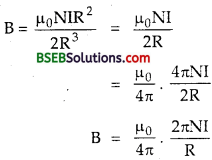which is the required familiar expression for the magnetic field at the centre of the coil.

(b) Let R be the radius of each coaxial circular coils. N = number of turns in each coil. R = distance between the two coils = CD. Let 2d < Rbe the small distance around the mid-point of CD i.e. O, where we want to show that the field is uniform.

Let I = Current flowing through the two coils in the same direction. We know that the magnetic field at a point on the axis of the coil at a distance x from its centre is given byLet B1 and B2 be the magnetic fields at point D’ due to the coils (1) and (2) respectively on their axes. Putting x = 𝑅2 + d for (1) coil and x = 𝑅2 – d for coil (2) in equation (i), we getIf B be the total magnetic field at P due to the two coils, then B = B1 + B2 B1 and B2 are added up because both act in the same direction.)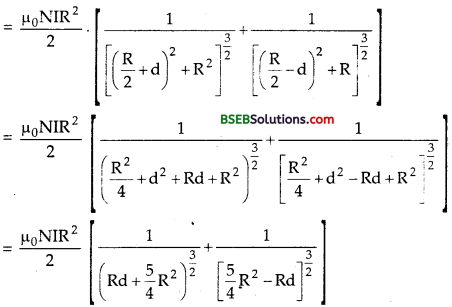Meglecting d2 and d2<<R2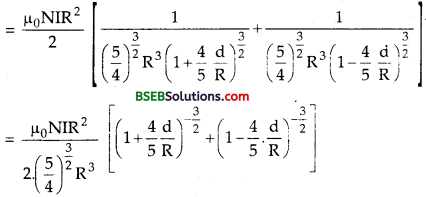Using Binomial expression theorem, we get= 0.72. 𝜇0NIR which is independent of the small distance and hence is uniform. Hence proved.

Question 17. A toroid has a core (non-ferromagnetic) of inner radius 25 cm and outer radius 26 cm, around which 3500 turns of a wire are wound. If the current in the wire is 11 A, what is the magnetic field (a) outside the toroid, (b) inside the core of the toroid, and (c) in the empty space surrounded by the toroid. Answer: Here, inner radius r1 = 25 cm = 25 x 10-2 m outer radius, r2 = 26 cm = 26 x 10-2 m.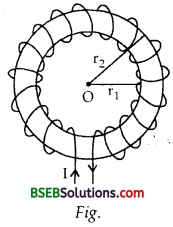N = Totahnumber of turns = 3500 I = Current in the wire = 11 A Let r be the mean radius of the toroid ∴ r = 𝑟1+𝑟22 = (25+26)×10−22 = 25.5 x 10-2 m. Total length of the toroid = circumference of the toroid = 2πr = 2 x π x 25.5 x 10-2 m = 51 π x 10-2 m If n be the number of turns per unit length of toroid, then n = 𝑁2π𝑟 = 350051𝜋×10−2 m−1 (a) Let B0 be the magnetic field outside the toroid = ? The field is non-zero only inside the core surrounded by the windings of the toroid, so the magnetic field outside the toroid is zero i.e B0 = 0

(b) Let B1 be the magnetic field inside the core of the toroid =? B1 = μ0 nI = 4π x 107 x 350051𝜋×10−2 x 11 = 0.0302 T = 3.02 x 10-2 T

(c) The magnetic field in the empty space surrounded by the toroid is also zero as the field is non zero only inside the core surrounded by the windings of the toroid.

Question 18. Answer the following questions (a) A magnetic field that varies in magnitude from point to point but has a constant direction (east to west) is set up in a chamber. A charged particle enters the chamber and travels undeflected along a straight path with constant speed. What can you say about the initial velocity of the particle?

(b) A charged particle enters an environment of a strong and non-uniform magnetic field varying from point to point both in magnitude and direction, and comes out of it following a complicated trajectory. Would its final speed equal the initial speed if it suffered no collisions with the environment?

(c) An electron travelling west to east enters a chamber having a uniform electrostatic field in north to south direction. Specify the direction in which a uniform magnetic field should be set up to prevent the electron from deflecting from its straight line path. Answer: (a) We know that the force on a charged particle moving inside the magnetic field is given by : F→ = q (𝑣⃗ ×B→) The charged particle will travel undeflected i.e. the force on it will be zero if 𝑣⃗ ×B→ is equal to zero. 𝑣⃗ ×B→ can be zero either the initial velocity υ→ is parallel or antiparallel to B→

(b) Yes, its final speed would be equal to the initial speed if it suffered no collision with the environment. This,is because the magnetic field exerts force on the charged particle which is always perpendicular to its motion, so this force can change the direction of motion of the velocity υ→ and not its magnitude.

(c) The electrostatic field is directed towards south. Since the electron is negativity charged particle, therefore the electrostatic field shall exert a force directed towards north. So, if the electron is to be prevented from deflection from straight path, the magnetic force on the electron should be directed towards south. As the velocity υ→ of the electron is from west to east, the expression for the magnetic Lorentz force i.e. F→ m = – e (𝑣⃗ ×B→) tells us that the magnetic field B→ should be applied along the vertical and in the downward direction.

Question 19. An electron emitted by a heated cathode and accelerated through a potential difference of 2.0 kV, enters a region with uniform magnetic field of 0.15 T. Determine the trajectory of the electron if the field (a) is transverse to its initial velocity, (b) makes an angle of 30° with the initial velocity. Answer: Here, υ = potential difference = 2.0 kV = 2000 V, B = uniform magnetic field = 0.15 T Let e, m be the charge and mass of the electron. Also let υ be the velocity acquired by the electron when accelerated under potential difference V.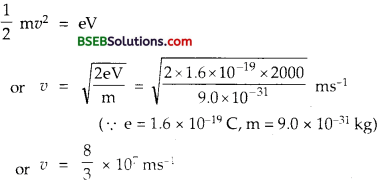(a) When the magnetic field is transverse to the initial velocity. The force on the electron due to transverse magnetic field =Be υ.This force provides the necessary centrepetal force to the electron required for uniform circular motion. So the trajectory of the electrons is circular having radius r (say) obtained as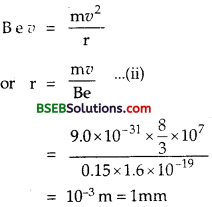(b) When the magnetic field makes an anele 30° with the initial velocity. Here θ = 30° ∴ Component of v nonnal to B→ is υ2 = υ sin 30° 12 or υ2 = 83 x 107 x 12 = 43 x 107 ms-1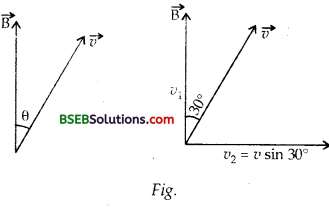Due to υ2, the force acting on the electron due to B→ will be providing the required centrepetal force, hence the electron will move along a circle.is the component of velocity along B→ , so no force will act on the electron due to vy So the electron shall follow a straight path along B→ Due to these two component velocities, the electron will describe a helical path having radius, r1→ (say) given by r1→ = m𝑣2Be (∴ υ2 = υ1 = component of υ→ normal to B→ = 9.0×10−31×43×1070.15×1.6×10−19 = 12 mm = 0.5 mm

Question 20. A magnetic field set up using Helmholtz coils (described in Exercise 4.16) is uniform in a small region and has a magnitude of 0.75 T. In the same region, a uniform electrostatic field is maintained in a direction normal to the common axis of the coils. A narrow beam of (single species) charged particles all accelerated through 15 kV enters this region in a direction perpendicular to both the axis of the coils and the electrostatic field. If the beam remains undeflected when the electrostatic field is 9.0 x 10+5 Vm”1, make a simple guess as to what the beam contains. Why is the answer not unique? Answer: Here, B = magnetic field =0.75 T E = Electric field applied normal to the axis of coils in the same region of B = 9 x 10-5 Vm-1 V = P.D. applied = 15 kV = 15000 V. Let e, m be the charge and mass of the charged particle. As the beam of charged particles remains undeflected, so the force due to magnetic and electric fields must be equal and opposite i.e. Fm = Fe or BeV = eE or υ = 𝐸𝐵 = 9×10+50.75 = 12 x 10+6 ms-1 nature of particles in the beam. Also 12 mυ2 = eV or 𝑒𝑚 = 𝑣22 V = (12×106)22×15000 = 4.8 x 107 C kg-1 Also, we know that 𝑒𝑚 for proton is = 9.6 x 10-7 x C kg-1. It follows m that the charged particle under reference has the value of 𝑒𝑚 half of that for the proton, so its mass is clearly double the mass of proton. Thus the beam may be of deutrons. The answer is not unique as the ratio of charge to mass i.e. 4.8 = 107 C kg-1 may be satisfied by many other charged particles, such as He++ (2𝑒2𝑚) and Li+++ (3𝑒3𝑚) which have the same value of (𝑒𝑚)

Question 21. A straight horizontal conducting rod of length 0.45 m and mass 60 g is suspended by two vertical wires at its ends. A current of 5.0 A is set up in the rod through the wires. (a) What magnetic field should be set up normal to the conductor in order that the tension in the wires is zero? (b) What will be total tension in the wires if the direction of current is reversed keeping the magnetic field same as before? (Ignore the mass of the wires.) g = 9.8m s2A Answer: Here, l = length of rod = 0.45 m. m = mass of rod = 60 g = 60 x 10-3 kg. I = current passing through the rod 5.0 A

(a) Tire tension in the wire will be zero if the force on Jhe wire due to the magnetic field is equal and opposite to the weight of the rod and is in the vertically upward direction. i.e BI l = mg B = mgI𝑙=60×10−3×9.85×0.45 = 0.261 T The force due to B→ will be upward if the direction of the field is horizontal and normal to the conductor as can be determined by applying Fleming’s left hand rule.

(b) When the direction of the current is reversed, then the force on the rod due to B will act in the direction of its weight i.e. vertically downwards, so tension in the supporting wire will be given by, T = BI l + mg = 0.261 x 5 x 0.45 + 60 x 10-3 x 9.8 = 0.588+ 0.588 = 1.176 N. i.e. T = mg + mg = 2 x weight of the rod.

Question 22. The wires which connect the battery of an automoblie to its starting motor carry a current of 300 A (for a short time). What is the force per unit length between the wires if they are 70 cm long and 1.5 cm apart? Is the force attractive of repulsive? Answer: Here, I1 = I2 = 300 A r = 1.5 cm = 1.5 x 102m Length of each wire, l = 70 cm = 70 x 10-2 m. We know that the force per unit length of a current carrying long conductor is given by F = 𝜇04𝜋×2I1I2r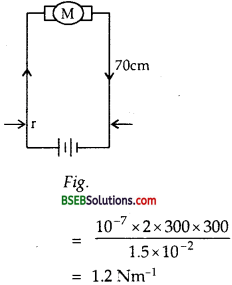As the current in the two wires connecting the battery to the starting motor flows in opposite directions, so the force is repulsive.

Question 23. A uniform magnetic field of 1.5 T exists in a cylindrical region of radius 10.0 cm, its direction parallel to the axis along east to west. A wire carrying current of 7.0 A in the north to south direction passes through this region. What is the magnitude and direction of the force on the wire if, (a) the wire intersects the axis (b) the wire is turned from N-S to northeast-northwest direction, (c) the wire in the N-S direction is lowered from the axis by a distance of 6.0 cm? Answer: Here, B = uniform magnetic field = 1.5 T. I = current in the wire = 7.0 A (a) Length of the wire in a cylindrical magnetic field is equal to the diameter of the cylinderical region i.e. Z = 2r = 2 x 10 = 20 cm = 20 x 10-2 m. Angle between the current and the magneitc field, 0 = 90° ∴ Force on the wire is given by F = BI l sin θ = 1.5 x 7.0 x 20 x 10-2 x sin 90 = 2.1 N The direction of this force is vertically downwards using Fleming’s left hand rule.

(b) When the wire is turned from N-S to north east – north west direction, then θ = 45° and length of the wire in the cylindrical region of the magneitc field is I1 (say) given by I = I1 sin 45° or I1 = 𝑙sin45∘ or I1 = 𝑙1/2√ = 2‾√ l If F1 be the force on the wire in this case, then F1 = B I l1 sin 45° = 1.5 x 7.0 x 2‾√ l x 𝑙1/2√ = 10.5 x 0.2 = 2.1 N = 2.1 N The force acts vertically downwards.

(c) When the wire is lowered by-6.0 cm i.e. taken at position CD as shown in the figure here, then the length of the wire in magnetic field is given byl2 = 2x where x is given by x.x = 4 x (10 + 6) = 64 or x2 = 64 x.x = 8cm. l2 = 2 x 8 = 16 cm = 16 x 10-2 Let F2 be the force on the wire in this case, F2 = BI l2 = 1.5 x 7x 10 x 10-2 = 1.68 N and it acts vertically downwards.

Question 24. A uniform magentic field of 3000 G is established along the positive z-direction. A reactangular loop of sides 10 cm and 5 cm carries a current of 12 A. What is the torque on the loop in the different cases shown in Fig. What is the force on each case? Which case corresponds to stable equlibrium ?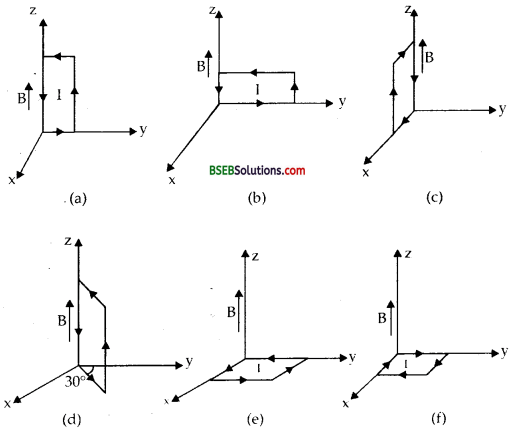answer: Here, B = unifrom megnetic feild gasus along z-axis = 3000 x 10-4 T = 0.3 T l = length of rectangular loop = 10 cm = 0.1 m b = breath of rectangular loop = 5 cm = 0.05 m ∴ A = area of rectangular loop = l x b = 10 x 5 = 50 cm2 = 50 x 10-4 m2 Torque on the loop is given by τ = IAB cos θ When θ is the angle between the plane of the loop and the direction of magnetic field. (a) Here θ = 0 τ = 0.3 x 12 x 50 x 10-4 x cos 0 = 1.8 x 102 Nm and acts along y’- axis i.e. – y direction

(b) θ = 0 τ = 0.3 x 12 x 50 x 10 x cos 0 = 1.8 x 102 Nm and acts along y’-axis or y-axis

(c) θ = 0 ∴ τ = 0.3 x 12 x 50 x 10 x cos 0 = 1.8 x 102 Nm and acts along – x direction or x’- axis

(d) Here θ = 0 ∴ τ = 1.8 x 10-2

(e) Here θ = 900, so cos 90 = 0 ∴ τ = BIA cos 90 = 0

(f) θ = 90 τ = 0

Aliter: τ→ = (A→)×B→ IA = 50 x 104 x 12 = 0.06 Am+2 (a) Here, I A→ = 0.06 î ,Am2, B→ = 0.3 k̂ T same as in case (a)

(c) I A→ = 0.06 î ,Am2, B→ = 0.3 k̂ T ∴ τ→ = (A→)×B→ = 0.06ĵ x 0.3 k̂ = -1.8 x 10-2 ĵ Nm ∴ τ = 1.8 x 10-2 Nm and acts along – x direction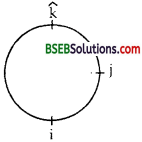(d) Here A→ = l→ x b→ = 0.1 k̂ x (0.05 cos 300 ĵ + 0.05 sin 30î ) = 5 x 10-3 3√2 k̂ x ĵ + 5 x 10-3 x 12k̂ x î = 2.5 x 10-3 (−3‾√𝑖̂ +𝑖̂ ) τ→ = (A→)×B→ = 12 x 2.5 x 10-3 (−3‾√𝑖̂ +𝑗̂ ) x 0.3 𝐤̂ = 9 x 10-3 (−3‾√𝑖̂ ×𝑘̂ +𝑗̂ ×𝑘⃗ ) = 9 x 10-3 $(\hat{i}-\sqrt{3}(-\hat{j})$ = 9 x 10-3(𝑖̂ +3‾√𝑗̂ ) Nm. ∴ magnitude of torque is given by τ→ is given by τ→ = (9×10−3)2+(9×10−3×3‾√)2‾‾‾‾‾‾‾‾‾‾‾‾‾‾‾‾‾‾‾‾‾‾‾‾‾‾‾‾‾‾√ = (9×10−3)2×(1+3)‾‾‾‾‾‾‾‾‾‾‾‾‾‾‾‾‾‾‾‾√ = (9×10−3×2)2‾‾‾‾‾‾‾‾‾‾‾‾‾‾‾√ = 18 x 10-3 = 1.8 x 10-2 Nm

Direction of τ→ : Let (1 be the angle made by τ→ with + ve direction of x-axis using A→ . B→ = AB cos θ, we get τ→ . î = (τ→) . (î ) cos β = τ . 1 . cos β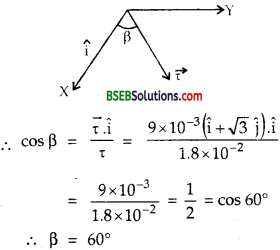(e) Here, IA→ = 0.06 k̂ Am2, B→ = 0.3 k̂ T τ→ = 0.06 k̂ x 0.3 k̂ = 0

(f) Here, IA→ = -0.06 k̂ Am2, IB→ = 0.3 k̂ T ∴ τ→ = -0.06 x 0.3 k̂ x k̂ = 0 Net force on a planar loop in a magnetic field is always zero, so force is zero in each case. Case (e) corresponds to stable equilibrium as IA→ is aligned with B→ while A→ corresponds to unstable equilibrium as IA is antiparallel to B→

Question 25. A circular coil of 20 turns and radius 10 cm is placed in a uniform magnetic field of 0.10 T normal to the plane of the coil. If the current in the coil is 0.5 A, what is tjre (a) total torque on the coil, (b) total force on the coil, (c) average force on each electron in the coil due to the magnetic field? (The coil is made of copper wire of cross-sectional area 10-5 m2, and the free electron density in copper is given to be about 1029 m-3,) Answer: (a) Here, n = number of turns in the circular coil = 20 r = radius of the circular coil = 10 cm = 10 x 10-2 m. B = uniform magnetic field = 0.10 T θ = angle between B→ and normal to the plane of coil = 0° I = current in the coil = 5.0 A A = Area of the coil = πr2 = 227×(0.10)2 = m2 τ = torque on the coil = ?

(a) Using formula, τ = n B I A sin θ We get, τ = 20 x 5 x 0.10 x 227 x (0.10)2 x sin θ° = 0 (b) Total force on a planar current loop in a magnetic field is always zero. (c) Force on an electron is given by F = B e υd = Be (IneA′)=BInA (∴ I = ne A vd) Here, n = 1029 m-3 = free electron density in copper A’ = area of cross-section of copper wire = 10-5 m2 F = 0.10×51029×10−5 = 5 x 10-25 N

Question 26. A solenoid 60 cm long and of radius 4.0 cm has 3 layers of windings of 300 turns each. A 2.0 cm long wire of mass 2.5 g lies inside the solenoid (near its centre) normal to its axis; both the wire and the axis of the solenoid are in the horizontal plane. The wire is connected through two leads parallel to the axis of the solenoid to an external battery which supplies a current of 6.0 A in the wire. What value of current (with appropriate sense of circulation) in the windings of the solenoid can support the weight of the wire? g = 9.8 m s-2 Answer: Let I be the current passed through the solenoid. If B = the magnetic field set up at the centre of the solenoid, then B = μ0 nl ……..(i) This field acts normally to the length of the current carrying wire, thus the wire experiences a force F given by F = BI’ l …… (ii) When I’ = Current flowing through the wire = 6.0 A. and l = length of the wire placed inside the solenoid = 2 cm = 0.02 m Here, N = total no. of turns = no. of layers x no. of turns in each layer . = 3 x 300 = 900 L = length of solenoid = 60 cm = 0.6 m r = radius of solenoid 4 cm = 0.04 m n = 𝑁𝐿 = 1500 turns m-1 ∴ From (1) B = 4π x 10-7 x 1500 x I 6π x 10-4 I Tesla. 6π x 10-4 x 6 x 0.02 2.262 x 10-4 I N The direction of current through the solenoid and hence the magnetic field are understood to be in such a direction that the force F acts upwards and the wrightof wire will be supported only if F is equal and opposite to the weight of the wire i.e. F = mg or 2.262 x 10-4 I = mg m = 2.5 x 10-3 kg g = 9.5 ms-2 ∴ 2.262 x 10-4 I = 2.5 x 10-3 x 9.8 0r I = 2.5×10−3×9.82.262×10−4 = 108.3 A

Question 27. A galvanometer coil has a resistance of 12 Ω and the meter shows full scale deflection for a current of 3 mA. How will you convert the meter into a voltmeter of range 0 to 18 V? Answer: Here, G = galvanometer resistance = 12 Ω Ig = Galvanometer full scale deflection current = 3mA = 3 x 10-3 A V = 18V R = resistance to be connected in series for conversion of galvanometer into voltmeter of 0 -18 V range = ? Using the relation V = – Ig (R + G), we get R =VIg – G = 183×10−3 – 12 = 6000 – 12 = 5988 Ω

Question 28. A galvanometer coil has a resistance of 12 Ω and the meter shows full scale deflection for a current of 4 mA. How will you convert the meter into a voltmeter of range 0 to 6 V? Answer: Here, galvanometer resistance, G = 15 Ω Ig = current following in the galvanometer corresponding to which it gives full scale defection = 4 mA =4x 10-3 A I = total current to be measured by an ammeter = 6A. S = Shunt resistance to be connected in parallel to the galvanometer = ? Using the relation,### Bihar Board Class 12 Physics Chapter 4 Moving Charges and Magnetism Additional Important Questions and Answers

Question 1. State Biot-Savart’s law. Answer: It states that the magnetic field due to a small conductor of length dl, carrying a current I at a point P at a distance r from it it given by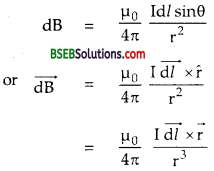Here θ is the angle between the direction of flow of current and the line joining the small conductor to the observation point.

Question 2. What type of magnetic field is produced due to a current carrying straight conductor? Answer: The magnetic field lines due to a current carrying straight condutor are the concentric circular loops lying in a plane perpendicular to the straight conductor with their centre on the conductor.

Question 3. What is the S.I. unit of permeability? Also write its dimensional formula. Answer: Tire S.I. unit of permeability is weber ampere-1 metre-1 (wb A-1 m-1) or tesla ampere-1 metre (TA-1 m). Tire dimensional formula of permeability is (MLT-2 A-2].

Question 4. In what respect does a wire carrying a current different from a wire carrying no current? Answer: A current carrying wire produces magnetic field because when the current flows through it, the electrons move inside it along a definite direction. On the otherhand, in a wire carrying no current, electrons are in random motion and thus such a wire producees no magnetic field.

Question 5. What is the force emperienced by a moving charge in a magnetic field. What force does a charge experience when it is stationary in the magnetic field? Answer: Tire force F→ on a charge q moving in a magnetic field B→ with a velocity υ→ is given by F→ = q( υ→ x B→ ) . or F = q υ B sin θ where θ is the angle made by υ→ with B→. When the charge is stationary, then force on it is is zero.

Question 6. State the principle of a moving coil galvanometer. Answer: It states that when a current carrying coil or loop is placed in a uniform magnetic field, it experiences a deflecting torque given by τ = NIAB sin θ = N MB sin θ where M = IA is the magnetic moment of the coil and 0 is the angle made by the normal to the plane of the coil with the direction of magnetic field.

Question 7. Equal currents are flowing through the two infinitely long parallel conductors. What shall be the magnetic field at a point mid way between them when the currents: (a) in them are flowing in the same direction? (b) in them are flowing in the opposite direction? Answer: The magnetic field at a point midway between two infinitely long parallel conductors shall be : (a) Zero when the currents in them are flowing in the same direction because magnetic fields due to these conductors are equal and opposite and thus cancel out each other. (b) Double the magnetic field due to one current carrying conductor because the magnetic fields due to these conductors are equal and act in the same direction.

Question 8. On what factors does the sensitivity of a moving coil galvanometer depends? Answer: It depends upon the following factors : (i) Number of turns of the coil N. (ii) Area of the coil. (iii) Magnetic field B→ . (iv) Restoring torque per unit twist for the material of the suspension wire i.e. k.

Question 9. What is the nature of the magnetic field in a movkig coil galvanometer and hence define it? Answer: The nature of the magnetic field in a moving coil galvanometer is radial magnetic field which is defined as the magnetic field parallel to the plane of the coil of the galvanometer.

Question 10. An electron or a proton is not deflected in passing through a certain region of space. Can we be sure that there is no magnetic field in that region? Why? Answer: No, we cannot be sure that there is no magnetic field in that region because an electron is not deflected even if it moves parallel or anti parallel to the magnetic field.

Question 11. An electron and a proton moving with the same speed enter the same magnetic field region at right angles to the direction of the field. For which of the two particles will be radius of the circular path be smaller? Answer: We know that Beυ = m𝑣2r or r = 𝑚𝑣𝐵𝑒 clearly r ∝ m As the mass of electron is lesser than that of the proton, so the radius of the circular path of the electron will be smaller.

Question 12. What are the primary function of electric and magnetic field in a cyclotron? Answer: The magnetic field makes the charged particle to cross the gap between the two dees again and again by making it to move along a circular path, while the oscillating electric field, applied across the dees accelerates the charged particle again and again.

Question 13. Mention the factors on which the magnitude of the force experienced by a charged particle depend? Answer: It depends upon the following factors :

• Magnitude of the charge q.
• Magnitude of the magnetic field in which the charge particle is moving.
• Magnitude of the velocity of the moving charge.
• Sine of the angle made by the velocity vector with the direction of the magnetic field.

Question 14. State Fleming’s Left hand rule. Answer: It states that if we stretch the thumb, first (fore) finger and central finger of our left hand in mutually perpendicular directions such that the first finger points in the direction of magnetic field, central finger points in the direction of electric current or velocity of the charge, then the thumb represents the direction of force experienced by the current carrying conductor (or the charged particle).

Question 15. Define magnetic field induction at a point in terms of the force experienced by a charge moving in a magnetic field and hence define one Tesla. Answer: Definition of B : We know that F = Bq υ sin q If. sin θ = 1 i.e., θ = 90°, υ = 1, q = 1, then F = B x 1 x 1 x 1 or B = F Thus B is defined to be numerically equal to the force experienced by a unit charge moving perpendicular to the magnetic field with unit velocity.

Definition of IT : If q = 1 C, υ = 1 ms-1 θ = 90°, F = 1 N, Then B = 1T ∴ 1T = 1 N C-1 m-1 s = 1 N-1 A-1 m-1. Thus the magnetic field at a’ point is said to be 1 T if 1 C charge moving perpendicular to the magnetic field with a velocity of 1 ms-1 experiences a force of 1 N at that point.

Question 16. State some important features of Biot-Savart’s law. Answer: Following are some important features of the law :

• It is applicable only to very small length current carrying conductors.
• This law can’t be easily verified experimentally.
• This law is analogous to Coulomb’s law in electrostatics.
•  It is valid for a symmetrical current distribution.
• The direction of dB is perpendicular to both I dl (current element) and r (position vector).
• dB = 0 on the axis of a thin linear current carrying conductor.
• dB = 0 i.e., minimum when 0 = 0 or 180° and maximum when 0 = 90°.

Question 17. What are the characteristics of Lorentz force? Answer: Following are the characteristics of Lorentz force. (i) Magnetic Lorentz force Fm−→ = q (υ→ x B→ ) always acts in a direction perpendicular to the plane containing υ→ and B→ (ii) Fm−→ does no work on the charge as it is always perpendicular to the direction of motion of the charge. (iii) Fm−→ on any charge q depends on the velocity of the charge as well as the magnetic field in which it is moving. (iv) Lorentz force due to a moving charge q in an electric field depends only on the strength of electric field artclis equal to q E→ . (v) Total force is given by F→ = Fe−→ + Fm−→ = q E→ + q (𝑣⃗ ×𝐵⃗ ) = q E→ + q (𝑣⃗ ×𝐵⃗ ) = q [E→ + (𝑣⃗ ×𝐵⃗ )]

Question 18. Why static magnetic field can’t change the kinetic energy of the moving charge? Answer: The force acting on a moving charge is always perpendicular to the magnetic field so static magnetic field cannot increase the force. Also the components of magnetic field in the direction of force is zero and the energy of moving charge is equal to the work done on it = Component of field along the direction of motion x charge = 0 x charge = 0.

Question 19. State right hand thumb rule. Answer: It states that if we hold a straight current carrying conductor in the palm of our right hand such that the thumb points in the direction of current in the conductor, then the curled fingers point in the direction of the magnetic lines of force (i.emagnetic field) produced around the conductor.

Question 20. Explain why the magnetic field falls of very rapidly irf case of the current carrying loop at a point on its axis? Answer: We know that the magnitude of the magnetic field at a point on the axis of the current carrying loop is given by B =𝜇0𝐼𝑅2𝑁2(𝑥2+𝑅2)3/2 When R = radius of loop, x = distance of the point from its centre. If x > > R, then R2 may be neglected in x2 + R2. B = 𝜇0IR2 N2x3=𝜇0IN2x3 or B ∝ 1x3 i.e., B varies inversely as the cube of the distance of the point from the centre of the loop, hence the field falls of very sharply with distance.

Question 21. State the principle of a moving coil galvanometer. Answer: It states that when a current carrying coil is placed or freely suspended in a uniform magnetic field, then the coil starts rotating due to the torque experienced by it. Magnitude of the torque acting on the coil depends on the current passed through the coil.

Question 22. Define the following: (a) Sensitivity of a galvanometer. (b) Current sensitivity of a galvanometer. (c) Voltage sensitivity of a galvanometer. Answer: (a) A galvanometer is said to be sensitive if it gives large deflection in its coil on passing a small current through it or on applying small voltage across its coil. (b) It is defined as the deflection produced in the coil of the galvanometer on passing unit current passed through it. 6 NAB i.e., Current sensitivity = 𝜃𝐼=𝑁𝐴𝐵𝑘 (c) It is defined as the deflection produced in the coil of a galvanometer on applying unit voltage across it. i.e., Voltage resistivity = 𝜃𝑉=𝜃𝐼𝑅=𝑁𝐴𝐵𝑘𝑅

Question 23. How will you identify whether the magnetic field at any point is due to earth or due to some current carrying conductor? Answer: If the magnetic field is due to earth’s magnetism only then the magnetic needle will always lie along the N-S direction On the other hand if the magnetic field is due to a current carrying conductor, then its direction may differ from N-S direction and it will come back to N-S direction when the current passing through the conductor is switched off.

Question 24. An electron beam is deflected in a given electric or magnetic field. How will you detect wether it has been deflected in electric or magnetic field? Answer: When the electron beam is passed through electric field or two parallel plates having a potential difference V across them, then the ‘ electrons are deflected towards the positive plate and the path of the electron beam is parabola. On the other hand, when the electron beam enters into a uniform magnetic field perpendicularly then its path is circular. If it enters at any . angle θ (≠ 0° and 180°), then the path will be helical.

Question 25. Answer the following questions: (a) Why does the picture of TV screen becomes distorted when a magnet is brought near the screen? (b) Why neutrons cannot be accelerated by a cyclotron? Answer: (a) The picture on TV screen becomes distorted when a magnet is brought near the screen because it exerts a magnetic force on the electron beam of T.V. tube. (b) Neutrons cannot be accelerated by a cyclotron because they are neutral.

Question 26. Define current element. What is its significance? Answer: It is defined as the product of current I and the length of the current dl→ . ∴ Current element = I dl→ Its significance is that it produces the magnetic field in fhe same way as a stationary charge produces an electric field.

Question 27. Is the source of magnetic field analogue to the source of electric field? Why? Answer: No, they are not analogue because source of the magnetic field cannot be a monopole whereas the source of electric field can be either of the positive or negative charges.

Question 28. When an ammeter is put in a circuit, does it read slightly less or more than the actual current in the original circuit? Explain. Answer: Ammeter reads slightly lesser than the actual currents when it is connected in a circuit because it has a small resistance which slightly decreases circuit current!

Question 29. Can we decrease the range of an ammeter? Why? Answer: We know that the shunt resistance is given by S = IgI−Ig . G S cannot be negative, so the range of ammeter cannot be decreased.

Question 30. What will be the nature of the path of a charged particle moving: (a) parallel to the magnetic field. (b) normal to the magnetic field. (c) at an arbitrary angle with the field direction. Answer: The path of the charged particle moving in a uniform magnetic field. (a) Parallel to B→ is a straight line. (b) Perpendicular to the B→ is circular. (c) At some angle with B→ is helical.

Question 31. How will you convert a moving coil galvanometer into: (a) an ammeter (b) a voltmeter Answer: A galvanometer is converted in to: (a) an ammeter by connecting a low resistance in parallel to the galvanometer. This low resistance is called shunt. (b) a voltmeter by connecting a high resistance in series with the galvanometer.

Question 32. Why should the ammeter have a low resistance? Answer: An ammeter should have a low resistance so that it may not change the resistance of the circuit when it is connected in series.

Question 33. What are the advantages of a moving coil galvanometer? Answer:

• It can be made extremely sensitive so as to detect even minute cyrrent in the circuit. –
• A linear scale can be used as the deflection of the coil of the galvanometer is directly proportional to the current.
• The external magnetic field cannot change the deflection of the coil of the galvanometer as the magnetic field is very strong.
• It is a dead beat type galvanometer which comes to the rest position quick by after disturbance.
• Moving coil galvanometer can be made ballistic by using a non-conducting frame instead of a metallic frame.

Question 34. What is the nature of the path of a charged particle moving in a uniform electrostatic field with initial velocity— (a) parallel to the field (b) perpendicular to the field (c) at an arbitrary angle with the direction of field. Answer: The path of the charged particle moving (a) parallel to the electric field is a straight line (b) perpendicular to the electric field is parabolic. (c) at an arbitrary angle with the direction of field is parabolic.

Question 35. Out of an ammeter and a voltmeter which of the two has a high resistance and why? Answer: Voltmeter has high resistance. This is because galvanometer is converted into voltmeter by connecting a high resistance in series with it.

Question 36. Why does a solenoid contract when current is passed through it? Answer: When the current is passed through a solenoid, the current in the adjacent turns of the solenoid flows in the same direction. We know that current flowing in the same direction between two conductors causes a force of attraction. So the turns of the solenoid will attract each other and hence the solenoid will contract.

Question 37. What is the magnetic field at the centre C of the following circular loop formed by sermi-circles APB and AQB? Why? Answer: Hie magnetic field at the centre of the circular loop given here is zero because the magnetic fields due to the two semicircles are equal in magnitude and opposite in direction.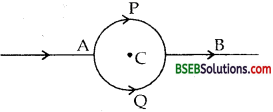Question 38. Compare a voltmeter and ammeter. Answer: (i) An ammeter is used to measure current while a voltmeter is used to measure the potential difference. (ii) An ammeter is a low resistance instrument while a voltmeter is a high resistance instrument. (iii) An ammeter is always connected in series while a voltmeter is always connected in parrallel.

Question 39. If a student by mistake connects a voltmeter in series with or an ammeter in parrallel or a circuit, what will happen? Answer: When the voltmeter is connected in series, the resistance of the circuit becomes high. Hence the current in the circuit will be reduced, so the voltmeter will not read the required potential difference. When the ammeter is connected in parallel in a circuit, the resistance is reduced considerably. So a large current would flow which can damage the ammeter.

Question 1. Calculate the magnetic field at the centre of a circular current carrying coil. Answer: Consider a circular loop of wire of radius r carrying a current 1 in the anticlockwise direction and lying in the plane of paper. Let n be the number of turns of the coil. Let A13 be a smalL current element I dl→ on the ioop. If dB−→ be the magnetic field at the centre of the coil due to the current element, then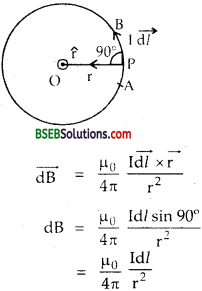If B be the total magnetic field at O due to the whole coil, then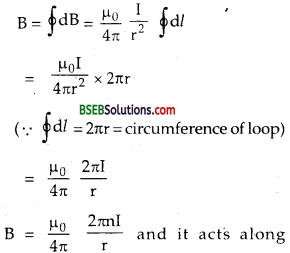perpendicular to te piane or paper towards us.

Question 2. Prove that dB−→ = 𝜇04𝜋d𝑙→×r→r3 for a current element. Answer: According to Bio-squarts law, we know that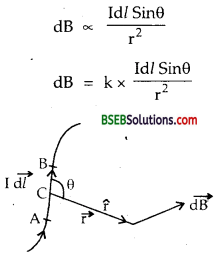When k is the proportionality constant and in S.I. system and in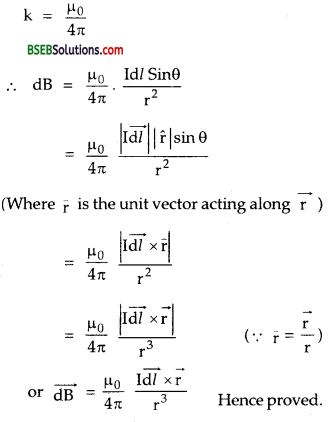Question 3. Derive the empression for the force on a current carrying conductor placed in a uniform magnetic field. Answer: Consider a conductor PQ of length 1 and area of cross reaction A lying in a uniform magnetic field acting along Z ‘axis. Let I be the current flowing in it along Y axis.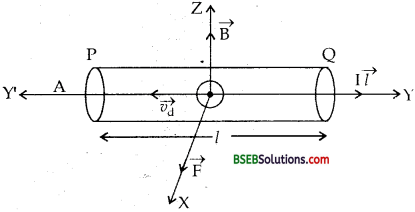I l→ = current element acting along y-axis. Let vd→ = drift velocity of free electrons along Y-axis n = no.of free electrons per unit volume. we know that I = neA υd ……. (i) Multiplying on both sides of eq (i) by 1, we get I l→ = neA l vd→ ……..(ii) As direction of conventional current is opposite to vd→ writing (ii) in vector form, we get I l→ = neAl vd→ …….(iii) Let f→ be the magnetic Lorentz force on the electron having charge -e, then f→ = -e(vd→ x B→ ) ……..(iv) Let N be the total number of electrons in the conductor ∴ N = carrier density x Volume of conductor = n x Al ……..(v) If F→ be the force on the current carrying conductor i.e. on N electrons in the conductor, Then F→ = N f→ = n A Z [- e (vd→ x B→)] (using (iv) and (v)) = -ne A l (vd→ x B→) = (-neAlvd→) x B→ F→ = I l→ x B→ (using (ii)) Clearly F→ acts along X-axis according to Fleming’s left hand rule. If θ be the angle between I l→ and B→ ,then the magnitude of F→ is given by F = BIl sin θ If θ = 90°, then Fmax =Bll.

Question 4. Derive the empression for the force on a charge moving in a magnetic field. Answer: Let q be a charge moving with a velocity υ→ in a magnetic field of strength B→ acting along Z – axis.Let θ be the angle made by υ→ with B→ If F be the force expericned by q, then it is found that the magnitude of the force (F) is : (i) directly proportional to q i.e. F ∝ q (ii) directly proportional to the magnitude of magnatic field F ∝ B. (iii) directly proportional to the component of the velocity normal to B→ i.e. F ∝υ sin θ combining, there factors, we get F ∝Bq υ sin θ or F = kB q υ sin θ When k is propertionality constant and in S.I. system, k = 1 . F = B q υ sin θ = q υ B sin θ or in vector form F→ = q (υ→ x B→ ) Tlaus F→ acts along X-axis is υ→ and B→ geting along Y and Z axes respectively as shown in fig, (2).

Question 5. Derive the empression for the force per unit length between two infinitely long parallel conductors and hence define one ampere current Answer: Let x1y1 and x2y2 be the two infinitely long parallel conductors lying in free space at a distance r in the plane of paper.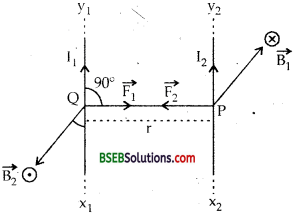I1, I2 = are the currents in them flowing in the same direction. If B→ be the magnetic field produced at P by x2y2 then B1 = 𝜇04𝜋2I1r ………(i) and acts along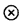i.e. ⊥ ar to the plane of paper and away from us. Now x2y2 is lying in B→ ⊥ arly, so it experiences a force per unit length F2−→ (say). F2 = B1I2l = 𝜇04𝜋2I1r I2 = 𝜇04𝜋2I1I2r ……….(iii) and it acts along PQ. Similarly, the magnetic field produced by x2y7 at Q (at the position of x1y1) is given by B2 = 𝜇04𝜋2I2r It acts along, i.e ⊥ ar to the plane of paper towards us. Now x1y1 lies perpendicular to B2 If F1be the force per unit length on it, then F1 = B2 I1l = 𝜇04𝜋2I2r l and it acts along QP. ∴ from (ii) and (iii) we see that F1 = – F2 i.e. the two conductors attract each other. or F1 = F2−→ = F = 𝜇04𝜋2I2r (Nm-1) Definition of IA : If I1 = I2 = IA, r = 1 m, then F = 2 x 10-7 Nm-1 Thus one Ampere is defined as that much current which when flows through each of the two parallel conductors of infinite length placed in free space 1m apart repel each other with a force of 2 x 10-7 Nm-1.

Question 6. State and explain Ampere’s circuital law. Answer: It states that the line integral of the magnatic field around a closed path is equal to go times the toal current I threading through the closed circuit, i.e. mathematically. ∮𝐵⃗ ⋅𝑑𝑙→ = μ0 I Proof : Let XY be a straight conductor lying in the plane of paper. Let I be the current flowing through it from X to Y. Let B→ be the magnetic field produced at a point P at a distance r from it. Then B = 𝜇04𝜋2𝐼𝑟 and acts alongaccording to right hand thumb rule.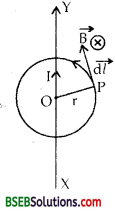Now consider a closed circular path of radius r. If dl→ be the small element of the path at P, then B. dl→ = B dl cos θ = B dl because B→ acts tangentially to the path. So the line integral of B→ around the closed path is given by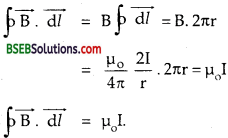Question 7. Show that if a charged particle moves in an electric field, the follows a parabolic path. Answer: Let V be the potential difference applied across the two parrallel plates P and Q st. P being at the +ve potential, d = distance between them.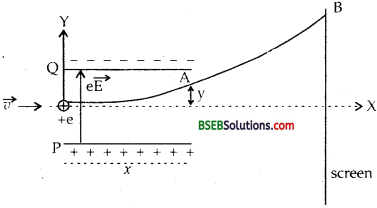Let +e be the charge entering the plates at point O with an initial velocity υ→ along X-axis. Let E→ = electric field set up between the plates. Thus a force +e E→ acts on the +e charge along P to Q. Let y = displacement of the charge at point A after a time t ∴ t = 𝑥υ ……….(1) and F→ = eE→ if a→ = acceleration produced in the charge along Y axis, Then a→ = 𝐹⃗ 𝑚=𝑒𝐸⃗ 𝑚 initial velocity along Y axis = 0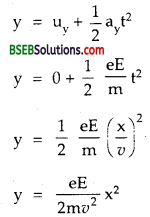Also we know that y = kx2 is the equation of a parabola. Hence the charged particle follows a parabolic path in E→

Question 8. State principle of cyclotron. What is its use and theory. Ans. It states that when a charged particle enters in a perpendicular magnetic field, it moves along a circular path of a difinite radius. If high frequency electric field is also applied, then path of the charged particles becomes spiral of larger and larger radius and its energy goes on increasing with the increase in its speed. It is used to accelerate positively charged particles i.e. proton, a particle etc. Theory: The magnetic Lorentz force Be v provides the necessary centrepetal force F = m𝑣2r or r = m𝑣Be Where m, v, e are the mass, velocity and charge of the particle to be accelerated. B = uniform magnetic field.

Question 9. Derive the expression for time period and the maximum kinetic energy gained by the ion inside the cyclotron. Answer: Let T be the time taken to complete one revolution ∴ T = 2𝜋𝑟𝑣=2𝜋𝑣⋅𝑚𝑣𝐵𝑒=2𝜋𝑚𝐵𝑒

Max K.E. : Let n be the number of revolutions of the ion inside the cyclotron, so it crosses the dees 2n times. Let V = potential difference between the dees, e = charge on postitive ion. ∴ energy gained by the ions per crossing of dees Emax = eV. If Emax be the maximum energy gained for 2 n times crossing, then Emax = 2nEmax = 2n. eV Also let υmax be the maximum velocity attained by the ions in a path of maximum radius rmax.Question 10. What is a galvanometer? Why it cannot read strong currents? Answer: Galvanometer is an instrument to measure weak currents of the order of milli amperes. It is quite sensitive instrument and gives large deflection for weak currents and its resistance is also small. If a strong current is passed through a galvanometer it would produce a very large defelection which would be unmeasurable. Strong currents may bum coil by producing large heat (I2R).

Question 11. How can galvanometer be converted into an ammeter of different range? What is the resistance of the ammeter and value of S? Answer: Galvanometer can be converted into an ammeter by using a shunt of an appropriate value. Shunt is a small resistance always connected in parallel to the instrument. A large amount of the main current passed through the galvanometer passes through the shunt and a very small current passes through the galvanometer.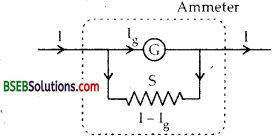Let I be the main current to be measured. G = resistance of the gavanometer, S = resistance of shunt. Ig = current passing through the galvanometer. I – Ig = current passing through the shunt. Now as G and S are connected in parallel, so P.D. across G = P.D. across S or IgG = (I – Ig) S ∴ S = IgI−Ig G Let RA be the ammeter resistance. ∴ 1𝑅𝐴=1𝐺+1𝑆=𝐺+𝑆𝐺𝑆 ∴ RA = 𝐺𝑆𝐺+𝑆

Question 12. How a galvanometer can be converted into voltmeter? Answer: To convert a glavenometer into voltmeter, we connect a high resistanceR in series with the given galvanometer and the value of R depends on the voltage range of the voltmeter to be measured.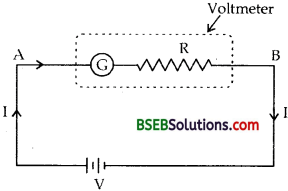Let V be the voltage to be measured. a = galvanometer renistance. If Ig be the maximum current which can be passed thorugh the galvanometer, then P.D. across points A and B is given by V = Ig (G + R) or R = VIG−G If Rv be the voltmeter resistance, then Rv = G + R.

Question 13. Derive the impression for the magnetic field due to a toroidal solenoid. Answer: A solenoid in the form of a ring is called toroidal solenoid. Let r be the radius of the ring of centre O. Let n be the number of turns per unit length of the solenoid^ wound on the ring.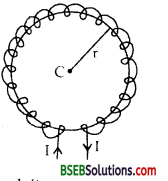I = current passed through it. The magnetic field produced on the circumference of the ring will be same and acts along the tangent to the ring. ∴ According to Ampere’s circuital law, ∮B→⋅d𝑙→ = µ0I x (total current threading the ring) = µ0I ……..(i) where I’ = total current threading the ring. Now number of turns in the solenoid of radius r = 2π r n. ∴ total current threading the ring of radius r is I’ = (2πrn)I …….(ii) From (i) ancf(ii), we get ∮B→⋅d𝑙→ = µ0 x 2πrnl or B. 2 π r = µ0 x 2 πrnl or B = µ0nI.

Question 14. Dervie an expression for the magnetic field Answer: XY is straight conductor carrying a current I from X to Y. a = ⊥ ar distance of the point P from the conductor at which B→ is to be calculated. AB = current element = I dl→, r = AP If dB be the magnetic field at P due to I dl→, ThenDraw AN perpendicular to BP. Let ∠MPA = ø, ∠APB = dø AN = rdø ………(ii) Also in ∆ABN, AN = AB sin θ = dl sin θ ……..(iii) from (ii) and (iii), we get dl sin θ = rd ø ………(iv) from (i) to (iv),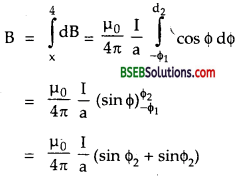or dB = 𝜇04𝜋Id𝜙r ….(v) Also in rt. angled ∆AMP cos ø = 𝑎𝑟 or 1𝑟 = 𝑐𝑜𝑠ø𝑎 ……(vi) from (v) and (vi), we get dB = 𝜇04𝜋Ia cos ø dø If B be the total magnetic field at P due to the whole conductor XY, then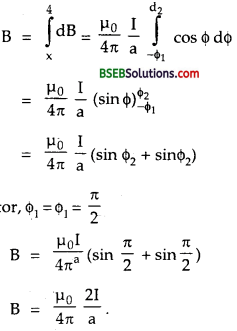for infinitely long conductor, ø1 = ø1 = π2 B = 𝜇0I4𝜋a(sin𝜋2+sin𝜋2) B = 𝜇04𝜋2𝐼𝑎

Question 15. Derive the expression for magnetic field due to a straight solenoid at a point inside it. Answer: Let n be the number of turns per unit length of the solenoid.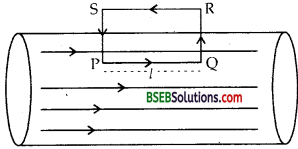Let I = current flowing through the solenoid i.e. through each turn. Let us now calculate the magnetic field at a point P inside the solenoid. Consider a rectangular path PQRS of length 1 and breadth b. Let B→ be the magnetic field at P. line integral of B→ around the closed path PQRS is given by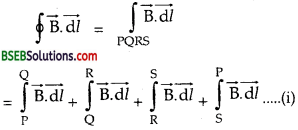Now as B→ is parallel to PQ, but normal to QR and SP and outside the solenoid, ∴ from (i), ∫B⋅d𝑙−→−−=∫2PB→⋅d𝑙→+0+0+0 = ∫QPB⋅d𝑙−→−−=B𝑙 …….(ii) Let I1= Total current threading the coil of length l. Then, I1 = nl I Now according to Amperes circuital law. ∫B→⋅d→𝑙=𝜇oI1 = μ0 I1 or B l = μ0n lI or B= μ0nl which is the required expression of B→

Question 1. For a circular coil of radius R and N turns carrying current the magnitude of the magnetic field at a point on its axis at a distance X from its centre is given by B = 𝜇0IR2 N2(x2+R2)3/2 (a) show that this reduces to the familiar result for field at centre of coil.Answer: Consider a circular coil of N turns carrying curent I in anticlockwise direction and held perpendicular to plane of paper. Suppose R is radius of coil and P is a point at a distance X from centre O, where we have to calculate B. Consider one turn of coil and we can suppose it to be made up of large number of elements. Magnetic field at

P will be sum of magnetic fields due to all elements. Consider two elements KL and MN with centre O’ and O” of length dl diametrically opposite to each other. Applying Biot Savarfs law magnetic field at P due current element KL is given by (dB1 say): dB1 = 𝜇04𝜋×𝐼 d𝑙sin𝜃(X2+R2)

Here θ = 90° direction of dB is along PS. dB1 = 𝜇04𝜋×𝐼 d𝑙(𝑋2+𝑅2) (along PS) Simlarly dB2 magnetic field due to current element MN at P is given by dB2 = 𝜇04𝜋×𝐼 d𝑙𝑋2+𝑅2 (along PT) dB1 dB2 = dB (say) dB1 and dB2 along PS and PT can be resolved into two rectangular components. dB cos θ along PU and PV both being opposite cancel. dB sin θ both along PW add up. Magnetic field at P due to a current element of length dl = dB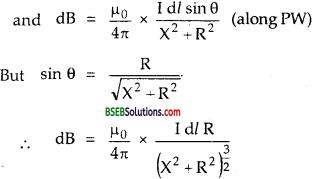Magnetic field at P due to whole coil is given by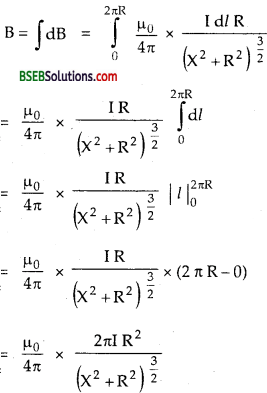if coil has N turns, then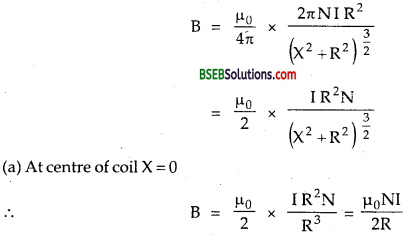Which is very familiar result.

Numerical Problems

Question 1. A’ flat circular coil of average radius 3 cm has 100 turns. It is placed in the plane of paper and a current of 3 A is passed in it in the anticlockwise direction as seen from above. Calculate the magnetic induction at: (a) the centre of the coil. (b) at a point on the axis of the coil at a distance of 4 cm from its centre. Answer: Here, a = 3 cm = 3 x 10-2 m N = 100 I = 3A x = 4 cm = 0.04 m

(a) B at centre = ? Using the formula, B = 𝜇0NII2a, we get(b) Using formula, B = 𝜇0NIa22(𝑎2+𝑥2)32, we getand it acts along the axis of coil in upward direction.

Question 2. Calculate the magnetic field at the centre of a coil in the form of a square of side 4 cm carrying a current of 5 A. Answer: Here, I = 5A flowing in the clockwise direction. AB = CD = BC = AD = 4 cm = 0.04 m. O is the centre of square where two diagonals intersect at right angles to each other. So ∠BOC = 90° or ∠BOM = ∠MOC = 45°. OM = a = perpendicular distance of the conductor BC from O, where B is to be calculated. Also OM = a = 42cm = 2 x 10-2 m. Let B1 be the field at O due to the conductor BC.Using this FormulaIf B be the total magnetic field at O due to the square, then B = 4 B1 = 4 x 3.54 x 10-5T = 1.42 x 10-4 T and it acts perpendicular to the plane of the loop and directed downwards.

Question 3. An electron is moving at 10s ms-1 in a direction parallel to a current of 5 A flowing through an infinitely long straight wire separated by a perpendicular distance of 10 cm in air. Calculate the magnitude of the force experienced by the electron. Answer: Here, q = 1.6 x 10-19 C, υ = 106 ms-1 I = 5A, Perpendicular distance = a – 10 cm = 0.10 m. F = force on the electron = ? Using formula, F = quB, where B = 𝜇0I2𝜋a, we get F = qυ. 𝜇0I2𝜋a = 1.6 x 10-19 x 106 x 4𝜋×10−72𝜋×50.10 = 1.6 x 10-18 N

Question 4. A wire of length 20 cm and mass 50 mg lies in a direction 300 east of south. The earth’s magnetic field at this point is horizontal and has a magnitude of 8.0 x 103 T. What current must be passed through the wire so that it may float in air ? (g = 10 ms-2). Answer: Here, m mass of wire = 50 mg =50 x 10-6 kg. g = 10 ms-2 B = 8.0 x 10-3T. l = 20 cm = 0.20 m. θ = 30°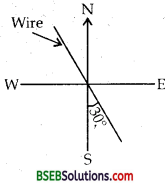Force acting on the current carrying wire in the magnetic field is F = BIl sin θ and acts vertically upward. The wire will float in air if the force on the wire due to B→ is balanced by the weight of the wire.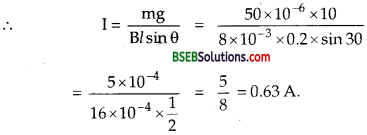Question 5. Compare the current sensitivity and voltage sensitivity in the following moving coil galvanometers: MeterA:N = 30, A =1.5 x 10-3m2,B = 0.25T,R = 20 Ω MeterB:N =35,A = 2.O x 10-3m2,B = 0.25T,R = 30 Ω Springs in the two meters have the same torsional constants. Answer: Current sensitivity, Is = 𝑁𝐵𝐾𝑘Question 6. A galvanometerof resistance 50 gives full scale deflectioi for a current of 0.05 A. Calculate the length of shunt wire required t convert the galvanometer into an ammeter of range 0.5 A. The diamete of shunt wire is 4 mm and its resistivity is 5 x 10-7 Ωm. Answer: Here, Ig = 0.05 A I = 0.5 A G = 50 Ω S = ? Using the formula,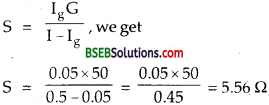l = length of shunt =? Also we know that S = R = ρ 1𝐴 = ρ = 𝑙𝜋𝑟2 or l = S𝜋r2𝜌 ……..(i) Here, D = 4mm =4 x 10-3 m p = 5 x 107 Ω m ∴ r = 𝐷2 = 2 x 103m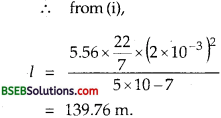Question 7. A galvanometer of 24Ω resistance can carry a full load of 500 μA. If it is shunted by a resistance of 3Ω, how much current can this system carry now without damage? Solution : Here, Ig = 500 μA = 500 x 10-6 A G = 24 Θ S = 3Ω I = ? Using formula, S = IgGI−Ig, we get I – Ig = IgGS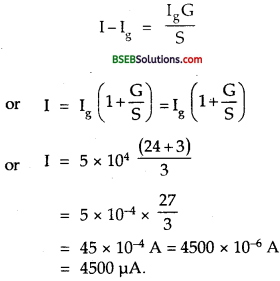Question 8. The current to be measured is arranged to go through two long parallel wires of equal lengths in opposite directions, one of which is linked to the point of current balance. The resulting repulsive form on the wire is balanced by placing a mass in the scale pan handing on the other side of the pivot. In one measurement, the mass in the scale pan 30g, the length of wires is 50 cm each and the separation between them is 10 mm. What is the value of the current being measured? Take g = 9.8 ms-2 and assume that the arms of the balance are equal. Solution: Let F’ = fore experienced per unit length I1 = I2 = I (say), r = separation between wires F = 𝜇04𝜋2I1I2r If F be the total force on wire of length l attached to the scale pan then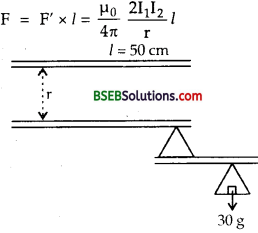When the pan is blanced, thenQuestion 9. A coil of radius 20 cm produces a magnetic field of 0.5 G at its centre when a current of 0.2 A flows through it. Find the number of turns the coil must have. Answer: Here, r = radius of coil = 20 cm = 0.20 m B = magnetic field produced at the centre of the coil = 0.5 G , = 0.5 x 10-4 T I = Current flowing in the coil = 0.2 A n = number of turns in the coil = ? Using formula,Question 10. An electron of kinetic energy 25 keV moves perpendicular to the direction of uniform magnetic field of 0.2 millitesla. Calculate the time period of rotation of the electron in the magnetic field. Solution: Here, B = magnetic field = 0.2 mT = 0.2 x 10-3 K.E. of Electron = 12 mu2 = 25 keV = 25 x 103 eV = 25 x 103 x 16 x 1019J T = time period of rotation of electron in the magnetic field = ? Using the relation,Question 11. A beam of protons with velocity 4 x 105 ms-1 enters a uniform magnetic field of 0.3 T at an angle of 60° to the magnetic field. Find the radius of the helical path taken by the proton beam. Also find the pitch of helix. Mass of Proton = 1.67 x 10″ 27 kg. Solution: Here, θ = 60° m = 1.67 x 10-27kg B = 0.3 T ‘ υ = 4 x 105 ms-1 e = 1.6 x 10-19 c r = radius helical path = ? p = pitch of helix = ? Using formula, r = 𝑚𝑣⊥𝑒𝐵 = m𝑣sin𝜃eB, we getQuestion 12. A rectangular loop of side 25 cm and 10 cm carrying a current of 15A is placed with its longer side parallel to a long straight conductor 2.0 cm apart carrying a current of 25A. What is the net force on the loop? Solution : Let XY be a straight wire and ABCD be a rectangular loop placed 2 cm a way from it as shown in figure.Here, AB = CD = 25 cm 0.25 m BC = DA = 10 cm = 0.10 m Let r1 and r2 be the distances of the arms AB and CD from XY ∴ r1 = 2 cm = 0.02 m and r2 = 2 + 10 = 12 cm – 0.12 m I1 = 15 A flowing in ABCD I2 = 25A flowing in XY The arm AB will get attracted while CD will get repelled and forces on BC and AD will cancel each other. if F1−→ and 𝜇04𝜋2I1I2r1 x leanth of AB = 10-7 x 2×15×250.02 x 0.25 = 9.375 x 10-4N towards XY and F2−→ = 𝜇04𝜋2I1I2r2 x length of CD 1.5625 x 10-4 N (a way from XY) i.e replusive if F→ be the net force on the loop then F→ = F1−→ – F2−→ = 9.375 x 10-4 – 1.5625 x 10-4 towards XY = 7.8125 x 10-4towards XY

Question 13. A voltmeter reads 5.0V at full scale deflection and is graded according to its resistance per volt at full scale deflection as 5000 ΩV-1. How will you convert it into a voltmeter that reads 20V at full scale deflection? Will it still be graded as 5000 ΩV-1. Will you prefer this voltmeter to one that is graded as 2000 ΩV-1? Solution: Here, resistance per volt at full scale deflection = 5000 ΩV-1 ∴ Currant for maximum deflection is given by Ig = 15000 A = 2 x 10-4 A (∴ Ig = resistance/volt at full scale deflection) Reading of volt meter at full scale deflection = 5 V If Rv be the voltmeter resistance, then Rv = 𝑉𝐼𝑔=52×10−4 = 2.5 x 104 Ω New range of voltmeter, V’ = 20V Let R be the resistance that should be connected in series so that voltmeter may read upto 20 V. R + Rv = V′Ig R = V′Ig – Rv = 202×10−4 – 2.5 x 104 = (10 – 2.5) x 10+4 If R’v be the resistance of the new voltmeter then R’v = R + Rv = 7.5 x 104 + 2.5 x 104 = 10 x 104 = 104 Ω ∴ Resistance per volt of the new voltmeter is = 202×10−4= 5000 ΩV-1 Yes, new voltmeter will be still graded as 5000 ΩV-1. A voltameter graded as 5000 ΩV-1 is better than one graded as 2000 ΩV-1. It is because, higher the resistance per volt lesser is the current it draws from the circuit and hence better it is.

Question 14. A test charge of 1.6 x 10-19C is moving with velocity υ→ = (2î + 3ĵ ) ms-1 in a magnetic field B→ =(lî + 3jĵ ) wb m2. Find the force acting on the test charge. Solution : Here,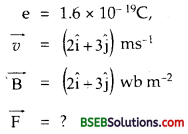Using Formula,i.e. no force acts on the test charge as it moves parallel to B→

Question 15. A beam of electrons is moving with a velocity of 3 x 106 ms-1 and carries a current of 1 pA. (a) How many electrons per second pass the given point? (b) How many electrons are in lm of the beam? (c) What is the total force on all the electrons in lm of the beam, if it passes through a field of 0.1 NA-1 m-1? (d) What is the force on a single electron, if the electrons experience the same force? Solution : Here, υ = 3 x 106 ms-1, 1 = 1 μA = 10-6 A

(a) The number of electrons passing a given point per second is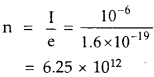(b) Since the electrons traverse a distance of 3 x 106 m in Is, the number of electrons in lm of the beam is given by: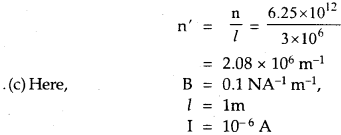∴ Force on lm of the beam of electrons is given by F = B I l = 0.1 x 10-6 x 1 = 10-7 N

(d) Since lm contains 2.08 x 106 electrons If f be the force on one electron, then F = n’fQuestion 16. In a cyclotron to accelerate proton, the magnetic field is 15000 gauss and radius of the dees is 15 cm. Calculate the energy of the proton beam and the frequency of the oscillator. Tabe e/m for proton to be 9580.84 e.m.u g’1 and mass of proton = 1.67 x 1024 g. Solution : Here, m = 1.67 x 10-24 g e/m = 9580.84 e.m.u. g-1 B – 15000 G r = radius of dees = 15 cm Emax = energy of the proton ? v = frequency = ? Using formula,Also using formula, v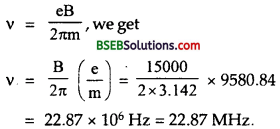Question 17. A current of 1.0 A is flowing in the sides of an equilateral triangle of side 4.5 x 10-2 m. Find the magnetic field at the control of the triangle, μ = 4π x 10-7 Vs A-1 m-1. Solution : Here, l = side of the equilateral triangle = 4.5 x 10-2 m I = Current flowing in the triangle .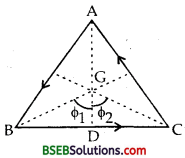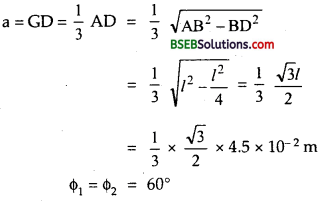If B1−→ be the magnetic field at G due to be current carrying conductor BC, then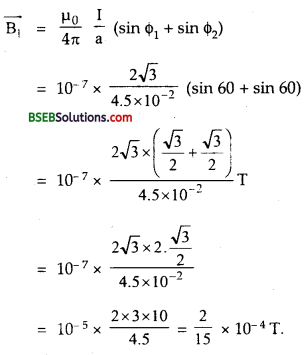As the field due to the three sides is in the same direction (i.e. perpendicular to the plane of triangle in upward direction), so the resultant field at a is B = 3B1 = 3 x 45 x 10-4 = 4 x 10-5 T

Question 18. A coil in the shape of an equilateral triangle of side 0.02 m is suspended from the vertex such that it is hanging in a vertical plane between the pole pieces of a permanent magnet producing a horizontal ,, magnetic field of 5 x 10-2t. Find the couple acting on the coil when a current of 0.1 A is passed through it and the magnetic field is parallel to its plane. Answer: Couple or Torque acting on the coil is given by x = BNAI sin θ Here, A = area of equilateral triangle = B1−→ a2 a = 0.02 m = side of equilateral triangle B = 5 x 10-2 T, θ = 90° ∴ τ = 5 x 10-2 x 1 x (0.02)2x 1 = 8.66 x 10-7Nm,

Question 19. An electron moves along a circular path of radius 2 x 10-10 m with a uniform speed of 3 x 106 ms-1. Find the magnetic field produced at the centre of the circular path. Also calculate the magnetic moment of the current loop. Solution : An electron moving in a circular path is equivalent to a circular current loop with current I = ne when n = no. of resolution/s = υ2π𝑟 1 = υ2π𝑟 e υ = 3 x 106, r = 2 x 10-10m, e = 1.6 x 1019 C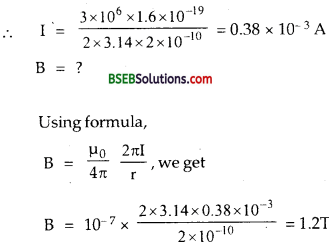Let M = Magnetic moment of the current loop =? … M = IA = 0.38 x 10-3 x πr2 = 0.38 x 10-3 x 3.14 x (2 x 10-10)2 = 4.77x 10-23 Am2.

Question 20. Given a uniform magnetic field of 100G in an east to west direction, and a 44 cm long wire with a current carrying capacity of at most 10A. What is the shape and orientation of the loop made of this wire which yields maximum turning effect on the loop? What is the magnitude of the maximum torque? Solustion : Here B = magnetic field = 100G = 100 x 10-4 T = 10-2 T length of wire – l = 44 cm = 0.44 m ‘ I = current carrying capacity of the wire = 10A We know that the torque on a current loop will be maximum if it has maximum area. For a given perimeter, a circle encloses maximum area. So the wire should be bent into a circular loop having radius V (say) given by 2πr = 0.04 0r r = 0.042𝑥22 x 7 = 0.07 m area of the circular loop A = πr2 = 227 x (0.07)2 = 154 x 10-4 m2 We know that torque on the coil is given by τ = BIA sin θ For τ to be maximum τmax sin θ = 1, τmax = BIA = 10-2 x 10 x 154 x 10-4 = 1.54 x 10-3Nm. Orientation of loop: From equation (1) τ = BIA sin θ where θ is the smaller angle between E→ and B→ For τmax , sin θ = 1 or θ = 90 which means that the plane of the coil is parallel to the direction of the magnetic field.

Fill In The Blanks

Question 1. The resistance of an ideal ammeter is and the resistance of an ideal voltmeter is ……. Answer: zero, infinite.

Question 2. The material used for the suspension wire of a moving coil galvanometer is ……. Answer: phosphor bronze.

Question 3. If μ0 and ∈0 be the magnetic permeability and electric permittivity of free space, p and e be the corresponding quantities for medium, then the refractive index of the medium in terms of these parameters is …… Answer: 𝜇∈𝜇0∈0‾‾‾‾‾√

Question 4. When the radius of a circular current carrying coil is doubled and the current in it is halved, then the magnetic dipole-moment of the coil becomes ……… the original value. Answer: double.

Question 5. When a particle of charge q and mass m moving with velocity v, enters a magnetic field B at right angles to the direction of the field, the particle takes a circular path of radius ………. and time period of its oscillation is ……. Answer: m𝑣qB,2𝜋mBq

Question 6. Newton/Amp-meter and Weber/metre2 are the units of same physical quantity i.e ……… Answer: magnetic field of induction or magnetic field.

Question 7. A positive particle enters the magnetic field B→ per pendicularly with a velocity υ→ The Lorentz force will act on it in a direction …….. Answer: pendicular to both B→ and υ→

Question 8. The sensitivity of a moving coil galvanometer can be increased by decreasing ………. Answer: The couple per unit twist (k) of the suspension wire.

Question 9. An electron enters a magnetic field whose direction is perpendicular to the velocity of the electron then the speed of the electron will ……… Answer: remain the same.

Question 10. A current carrying loop is placed in a uniform magnetic field. The torque acting on it does not depend upon Answer: the shape of the loop.

Question 11. To convert a galvanometer into an ammeter a ……… resistance is connected in ……… and to convert the galvanometer into a voltmeter a …….. resistance is connected in ……… Answer: low, parallel, high, series.

Question 12. A charged particle enters at 30° to the direction of magnetic field. Its path will be ………. Answer: Helical.

Question 13. Two parallel wires carrying current in the same direction attract each other because of ………. Answer: magnetic force between them.

Question 14. If a long copper rod carries a direct current, the magnetic field associated with the current will be …….. Answer: both inside and outside the rod.

Question 15. The magnetic effect of electric current was discovered by ……… Answer: Oersted.

Question 16. A current I flows along the length of an infinitely long, straight, thin wailed pipe, then the magnetic field at any point inside the pipe is ……… Answer: zero. [Hint: Because there are no charge carriers inside the pipe, hence the magnetic field at any point inside the pipe is zero],

Question 17. A current carrying conductor produces …….. Answer: Both the electric and the magnetic fields.

Question 18. If a current is passed through a vertical spring, the adjacent turns of the spring will ……… Answer: attract each other.

## Bihar Board Class 12th Physics Moving Charges and Magnetism Textbooks for Exam Preparations

Bihar Board Class 12th Physics Moving Charges and Magnetism Textbook Solutions can be of great help in your Bihar Board Class 12th Physics Moving Charges and Magnetism exam preparation. The BSEB STD 12th Physics Moving Charges and Magnetism Textbooks study material, used with the English medium textbooks, can help you complete the entire Class 12th Physics Moving Charges and Magnetism Books State Board syllabus with maximum efficiency.

## FAQs Regarding Bihar Board Class 12th Physics Moving Charges and Magnetism Textbook Solutions

#### Can we get a Bihar Board Book PDF for all Classes?

Yes you can get Bihar Board Text Book PDF for all classes using the links provided in the above article.

## Important Terms

Bihar Board Class 12th Physics Moving Charges and Magnetism, BSEB Class 12th Physics Moving Charges and Magnetism Textbooks, Bihar Board Class 12th Physics Moving Charges and Magnetism, Bihar Board Class 12th Physics Moving Charges and Magnetism Textbook solutions, BSEB Class 12th Physics Moving Charges and Magnetism Textbooks Solutions, Bihar Board STD 12th Physics Moving Charges and Magnetism, BSEB STD 12th Physics Moving Charges and Magnetism Textbooks, Bihar Board STD 12th Physics Moving Charges and Magnetism, Bihar Board STD 12th Physics Moving Charges and Magnetism Textbook solutions, BSEB STD 12th Physics Moving Charges and Magnetism Textbooks Solutions,
Share: